# Hyperbolic function

"Sech" redirects here. For other uses, see Sech (disambiguation).
"Sinh" redirects here. For the garment, see sinh (clothing).
"Hyperbolic curve" redirects here. For the geometric curve, see Hyperbola.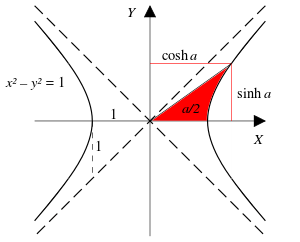A ray through the unit hyperbolain the point, whereis twice the area between the ray, the hyperbola, and the-axis. For points on the hyperbola below the-axis, the area is considered negative (see animated version with comparison with the trigonometric (circular) functions).

In mathematics, hyperbolic functions are analogs of the ordinary trigonometric, or circular functions.

The basic hyperbolic functions are the hyperbolic sine "sinh" (/ˈsɪn/ or /ˈʃn/), and the hyperbolic cosine "cosh" (/ˈkɒʃ/), from which are derived the hyperbolic tangent "tanh" (/ˈtæn/ or /ˈθæn/), hyperbolic cosecant "csch" or "cosech" (/ˈkʃɛk/ or /ˈksɛ/), hyperbolic secant "sech" (/ˈʃɛk/ or /ˈsɛ/), and hyperbolic cotangent "coth" (/ˈkθ/ or /ˈkɒθ/), corresponding to the derived trigonometric functions.

The inverse hyperbolic functions are the area hyperbolic sine "arsinh" (also called "asinh" or sometimes "arcsinh") and so on.

Just as the points (cos t, sin t) form a circle with a unit radius, the points (cosh t, sinh t) form the right half of the equilateral hyperbola. The hyperbolic functions take a real argument called a hyperbolic angle. The size of a hyperbolic angle is twice the area of its hyperbolic sector. The hyperbolic functions may be defined in terms of the legs of a right triangle covering this sector.

Hyperbolic functions occur in the solutions of many linear differential equations, for example the equation defining a catenary, of some cubic equations, in calculations of angles and distances in hyperbolic geometry and of Laplace's equation in Cartesian coordinates. Laplace's equations are important in many areas of physics, including electromagnetic theory, heat transfer, fluid dynamics, and special relativity.

In complex analysis, the hyperbolic functions arise as the imaginary parts of sine and cosine. When considered defined by a complex variable, the hyperbolic functions are rational functions of exponentials, and are hence holomorphic.

Hyperbolic functions were introduced in the 1760s independently by Vincenzo Riccati and Johann Heinrich Lambert. Riccati used Sc. and Cc. ([co]sinus circulare) to refer to circular functions and Sh. and Ch. ([co]sinus hyperbolico) to refer to hyperbolic functions. Lambert adopted the names but altered the abbreviations to what they are today. The abbreviations sh and ch are still used in some other languages, like French and Russian.

## Standard analytic expressions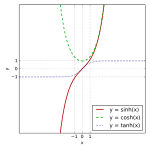sinh, cosh and tanh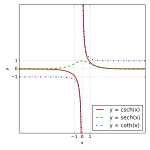csch, sech and coth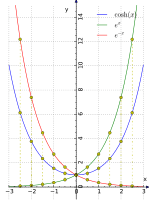(a) cosh(x) is the average of ex and e−x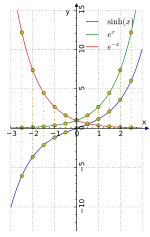(b) sinh(x) is half the difference of ex and e−x

The hyperbolic functions are:

• Hyperbolic sine:.
• Hyperbolic cosine:.
• Hyperbolic tangent:.
• Hyperbolic cotangent:• Hyperbolic secant:• Hyperbolic cosecant:Hyperbolic functions can be introduced via imaginary circular angles:

• Hyperbolic sine:• Hyperbolic cosine:• Hyperbolic tangent:• Hyperbolic cotangent:• Hyperbolic secant:• Hyperbolic cosecant:where i is the imaginary unit with the property that i2 = −1.

The complex forms in the definitions above derive from Euler's formula.

## Special meanings

### Hyperbolic cosine

It can be shown that the area under the curve of cosh (x) over a finite interval is always equal to the arc length corresponding to that interval:### Hyperbolic tangent

The hyperbolic tangent is the solution to the differential equationwith f(0)=0 and the nonlinear boundary value problem:## Useful relations

Odd and even functions:Hence:It can be seen that cosh x and sech x are even functions; the others are odd functions.Hyperbolic sine and cosine satisfy:the last which is similar to the Pythagorean trigonometric identity.

One also hasfor the other functions.

### Sums of argumentsparticularlyAlso:### Subtraction formulasAlso:Source.

### Half argument formulaswhere sgn is the sign function.If x ≠ 0, then## Inverse functions as logarithms## Derivativesif x ≠ 0if x ≠ 0if 1 < xif -1 < x < 1if x < -1 or 1 < xif 0 < x < 1if x ≠ 0

## Second derivatives

The second derivatives of sinh and cosh are the same function:All functions with this property are linear combinations of sinh and cosh, notably the exponential functionsand, and the zero function.

## Standard integralsThe following integrals can be proved using hyperbolic substitution:where C is the constant of integration.

## Taylor series expressions

It is possible to express the above functions as Taylor series:The function sinh x has a Taylor series expression with only odd exponents for x. Thus it is an odd function, that is, −sinh x = sinh(−x), and sinh 0 = 0.The function cosh x has a Taylor series expression with only even exponents for x. Thus it is an even function, that is, symmetric with respect to the y-axis. The sum of the sinh and cosh series is the infinite series expression of the exponential function.where:is the nth Bernoulli numberis the nth Euler number

## Comparison with circular functions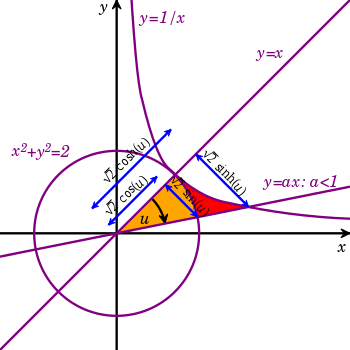Circle and hyperbola tangent at (1,1) display geometry of circular functions in terms of circular sector area u and hyperbolic functions depending on hyperbolic sector area u.

The hyperbolic functions represent an expansion of trigonometry beyond the circular functions. Both types depend on an argument, either circular angle or hyperbolic angle.

Since the area of a circular sector with radius r and angle u isit will be equal to u when r = square root of 2. In the diagram such a circle is tangent to the hyperbola xy = 1 at (1,1). The yellow sector depicts an area and angle magnitude. Similarly, the yellow and red sectors together depict an area and hyperbolic angle magnitude.

The legs of the two right triangles with hypotenuse on the ray defining the angles are of length √2 times the circular and hyperbolic functions.

Mellon Haskell of University of California, Berkeley described the basis of hyperbolic functions in areas of hyperbolic sectors in an 1895 article in Bulletin of the American Mathematical Society (see External links). He refers to the hyperbolic angle as an invariant measure with respect to the squeeze mapping just as circular angle is invariant under rotation.

## Identities

The hyperbolic functions satisfy many identities, all of them similar in form to the trigonometric identities. In fact, Osborn's rule states that one can convert any trigonometric identity into a hyperbolic identity by expanding it completely in terms of integral powers of sines and cosines, changing sine to sinh and cosine to cosh, and switching the sign of every term which contains a product of 2, 6, 10, 14, ... sinhs. This yields for example the addition theoremsthe "double argument formulas"and the "half-argument formulas"Note: This is equivalent to its circular counterpart multiplied by −1.Note: This corresponds to its circular counterpart.The derivative of sinh x is cosh x and the derivative of cosh x is sinh x; this is similar to trigonometric functions, albeit the sign is different (i.e., the derivative of cos x is −sin x).

The Gudermannian function gives a direct relationship between the circular functions and the hyperbolic ones that does not involve complex numbers.

The graph of the function a cosh(x/a) is the catenary, the curve formed by a uniform flexible chain hanging freely between two fixed points under uniform gravity.

## Relationship to the exponential function

From the definitions of the hyperbolic sine and cosine, we can derive the following identities:andThese expressions are analogous to the expressions for sine and cosine, based on Euler's formula, as sums of complex exponentials.

## Hyperbolic functions for complex numbers

Since the exponential function can be defined for any complex argument, we can extend the definitions of the hyperbolic functions also to complex arguments. The functions sinh z and cosh z are then holomorphic.

Relationships to ordinary trigonometric functions are given by Euler's formula for complex numbers:so:Thus, hyperbolic functions are periodic with respect to the imaginary component, with period(for hyperbolic tangent and cotangent).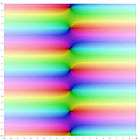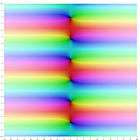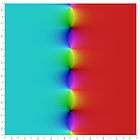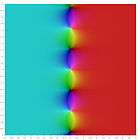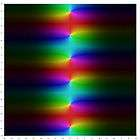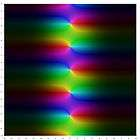Wikimedia Commons has media related to Hyperbolic functions.
1. (1999) Collins Concise Dictionary, 4th edition, HarperCollins, Glasgow, ISBN 0 00 472257 4, p.1386
2. Collins Concise Dictionary, p.328
3. Collins Concise Dictionary, p.1520
4. Collins Concise Dictionary, p.1340
5. Collins Concise Dictionary, p.329
6. tanh
7. Abramowitz, Milton; Stegun, Irene A., eds. (1972), Handbook of Mathematical Functions with Formulas, Graphs, and Mathematical Tables, New York: Dover Publications, ISBN 978-0-486-61272-0
8. Robert E. Bradley, Lawrence A. D'Antonio, Charles Edward Sandifer. Euler at 300: an appreciation. Mathematical Association of America, 2007. Page 100.
9. Georg F. Becker. Hyperbolic functions. Read Books, 1931. Page xlviii.
10. N.P., Bali (2005). Golden Integral Calculus. Firewall Media. p. 472. ISBN 81-7008-169-6.
11. Eric W. Weisstein. "Hyperbolic Tangent". MathWorld. Retrieved 2008-10-20.
12. "Derivation of tanh solution to 1/2 f′′=f^3−f...". Math StackExchange. Retrieved 18 March 2016.
13. Martin, George E. (1986). The foundations of geometry and the non-euclidean plane (1., corr. Springer ed.). New York: Springer-Verlag. p. 416. ISBN 3-540-90694-0.
14. "math.stackexchange.com/q/1565753/88985". StackExchange (mathematics). Retrieved 24 January 2016.
15. Osborn, G. (July 1902). "Mnemonic for hyperbolic formulae". The Mathematical Gazette. 2 (34): 189. JSTOR 3602492.
16. Peterson, John Charles (2003). Technical mathematics with calculus (3rd ed.). Cengage Learning. p. 1155. ISBN 0-7668-6189-9., Chapter 26, page 1155